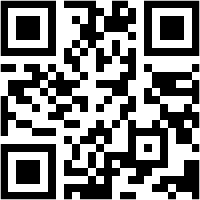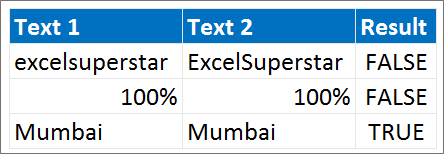Need Help? Chat with us

# Exact Function in Excel | Excel Formulas in Hindi

## Exact function in Excel

The Excel EXACT function compares two text string and returns TRUE if both the test strings are same and FALSE if both the strings do not match. The Exact function is an in-built function in Excel under String / Text function. It is a worksheet function so it can be entered as a part of the formula.

The Exact function was introduced in Excel in the year 2000. Exact function is case-sensitive. This article will answer to the question what is text string and how does this text string works? The Exact function helps us to do a comparison while we need to compare two value in the financial analysis.

## Special Offer for First Time Users

If you are the first time user and want to learn Microsoft office skills, then you can avail this special offer discount on your email.The Exact function requires two arguments. The arguments can be cell reference or can be typed manually.

The Syntax for Exact function is:

EXACT(text1, text2)

Where,

Text1: The first text string that we want to compare.

Text2: The second string that we want to compare.

NOTE: The equal sign in the formula is used to compare the text string. However, equal to sign is not case sensitive. (Text1=text2)

The below example will help you to clearly know how does Exact function work in Excel.Example 1

In the first example enter the following text in cell D4

=EXACT (B4, C4)

Result: False, because exact is case sensitive function which matches exact text strings. However here the both the strings do not match with each other. In the Example E and S are in the uppercase in cell C4, however in cell B4 it is lowercase.

Example 2

In the second example enter the following text in cell D5

=EXACT (B5, C5)

Result: False, because exact is a case sensitive function which matches text strings. However though it is seen that both the text string matches but the text string in cell C5 is rounded off due to the formatting conditions. So the result appear False.

Example 3

In the third example enter the following text in Cell D6

=EXACT (B6, C6)

Result: True. The result is true because both the text strings matches with each other and are exactly the same.

Note: If you change the format cell for the text string it will still return the result as TRUE. Since the formatting is changed.

Hope you like our tutorial for more cool and amazing trick of excel Like our Excel Superstar Facebook Page and subscribe to our Excel Superstar YouTube channel. Excel Superstar is the leading online training company, which provides Online Excel Course in Hindi. Connect with us and become an Excel Superstar.